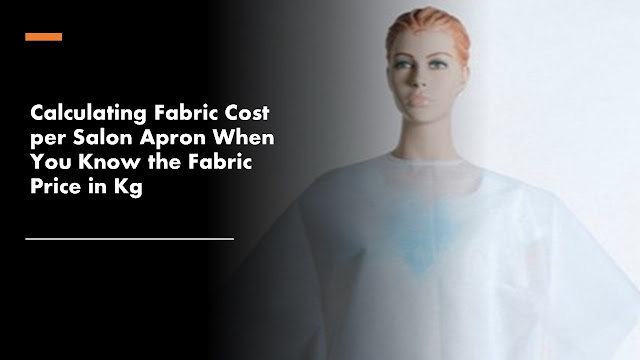# Calculating Fabric Cost per Apron When I Have Fabric Price in Kg

Question: I have a garment shop. I have started making and supplying salon aprons. A fabric roll has length 1000 meters, width 63 inches (1.6 meters). This fabric price is Rs. 126 per kg and the weight of the roll is 35kg. The size of the apron is 31.5" x 50" (0.8mtr x 1.3mtr). What would be the cost of 1 piece of apron? Note:-in 1.6 MTR width, I am to make 2 pic that is 0.8 MTR and length 1.3 meters.When you know the fabric price per Kg of fabric, you can covert the fabric price per meter using the method explained in this article.

In this question, the price of the apron fabric is given in Kg (weight) and you know the total weight of the fabric roll. So first we need to convert fabric price in meters. You have the fabric width and length in meters. With this information calculation of per apron fabric price will be easier.

The total price of the fabric roll @ Rs. 126/ per Kg x 35 Kg = Rs. 4410.00

You have mentioned that the fabric roll length is 1000 meters. So, we can say the price of 1000 meters roll is Rs. 4410.00
Therefore, per meter price = Rs. 4.410

You are making 2 aprons width-wise (apron size 1.3 meters x 0.8 meters)

Fabric cost of 2 apron pieces =(4.410 x 1.3)= Rs. 5.733

So, the cost of 1 piece apron would be = Rs. 5.733/2 = 2.87/-

Using the above steps you can calculate per piece fabric cost.